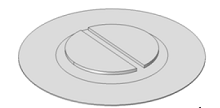# Technical Papers and Presentations

## Simulation and Experimental Characterizations of a Thin Touch Mode Capacitive Pressure Sensor

A.-M. El Guamra , D. Bühlmann , F. Moreillon , L. Vansteenkiste , P. Büchler , A. Stahel , P. Passeraub ,
 HES-SO University of Applied Sciences Western Switzerland, Delémont, Switzerland
 Institute for Surgical Technology & Biomechanics, University of Bern, Bern, Switzerland
 Bern University of Applied Sciences Engineering and Information Technology, Bern, Switzerland
Published in 2015

Introduction: This study describes a thin and low-cost capacitive pressure sensor in touch mode (TM) for monitoring fluid pressure from 0 to 40kPa in fluidic chambers with Luer fittings for medical applications. TM provides good linearity, large measuring range and large overload protection . The choice of a thin polymer membrane as sensitive element with printed circular electrodes reduces drastically the thickness and fabrication steps compared to standard microfabrication processes .

It was assumed that the real problem characterized by high-aspect ratio, mechanical contact and large deformations could be simplified .

Test bench: A simple disk was printed with a conductive ink on a 100μm thick diaphragm and fixed on the wall of the fluidic chambers in contact with fluid. A solid open capacitor with two half moon electrodes covered with a 16μm thick soldermask and dielectric constant of 4 was placed in front the conductive disk with a gap of 500μm. The geometry of the sensor is presented in figure 1.

The model: The model created using COMSOL Multiphysics® software couples a 2D-axisymmetric model to a 3D-model, which reduces computational and memory costs. The deflection is evaluated in 2D using the Structural Mechanics Module and this result is fed to the 3D-model via the general coupling operator.

It was assumed that the capacitance of the real system composed by the open capacitor with a 16μm contact gap, due to the soldermask's thickness, with the diaphragm is equivalent to a simpler system without soldermask and a 4μm contact gap. This solution avoids adding unnecessary complexity due to mechanical contacts and dielectric changes.

The ALE (arbitrary Lagrangian-Eulerian) method was used to enable displacement of the mesh with the diaphragm. Thus, the 3D-model couples sequentially the SME, the ALE method and the AC/DC Module to measure the capacitance.

Model validation: The deflection of the diaphragm without contact was measured experimentally with a 3D scanner and compared to the model. Analysis of figure 2a) shows that the simulation differs in average of 7% with respect to the experimental data.

The sensor was then simulated in TM to assess the response. The figures 2b) and 2c) show the deflections in cross section and the deflection of the central point. The capacitance was measured with a LCR-meter. The response is presented in figure 3. The latter is characterized by a 12pF near-linear measuring range and by a 2kPa linearity error. The regression line within the near-linear range gives a slope of 0.397 and 0.390 for the sensor and the model respectively. The standard deviations of y-residuals with the sensor regression line are 0.53pF and 0.56pF for the sensor and the model respectively. These results suggest good reliability of the model.

Conclusion: The model was validated by comparison with experimental data. It couples two models, two physics and the ALE method. Hypotheses have permitted to drastically simplify the real problem complexity and the response provides reliable predictions. The model may now be exploited for optimization purposes.